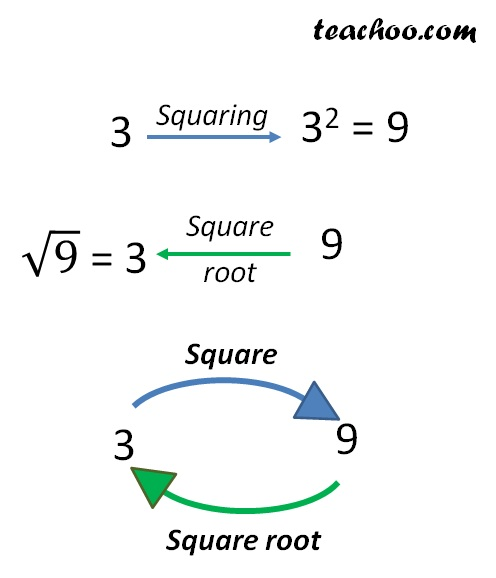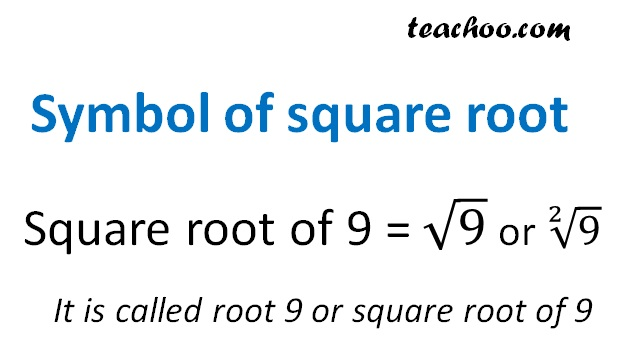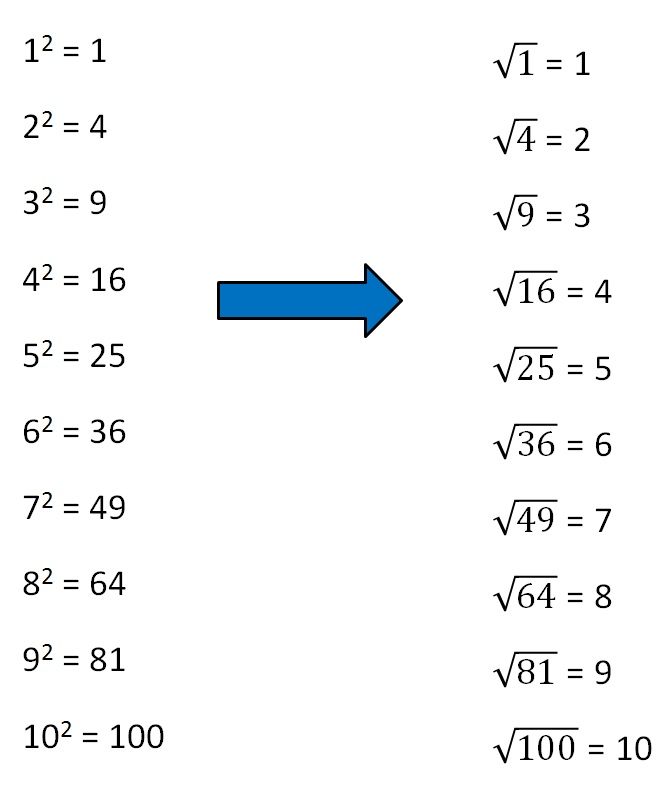Square root

Chapter 5 Class 8 Squares and Square Roots
Concept wise

Just like subtraction is the opposite of addition

Square root is the opposite of squaring

Number = 3

Square of number = 3 2

= 9

∴ Square root of 9 = 3

We write √9 = 3Let’s look at square roots of some number

Square Roots ExamplesLearn in your speed, with individual attention - Teachoo Maths 1-on-1 Class